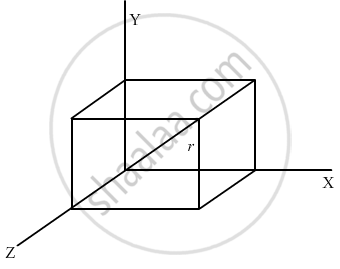Department of Pre-University Education, KarnatakaPUC Karnataka Science Class 11

# A Mosquito Net Over a 7 Ft × 4 Ft Bed is 3 Ft High. the Net Has a Hole at One Corner of the Bed Through Which a Mosquito Enters the Net. It Flies and Sits at the Diagonally Opposite - Physics

Sum

A mosquito net over a 7 ft × 4 ft bed is 3 ft high. The net has a hole at one corner of the bed through which a mosquito enters the net. It flies and sits at the diagonally opposite upper corner of the net. (a) Find the magnitude of the displacement of the mosquito. (b) Taking the hole as the origin, the length of the bed as the X-axis, it width as the Y axis, and vertically up as the Z-axis, write the components of the displacement vector.

#### Solution

Displacement vector of the mosquito,

$\vec{r} = 7 \hat {i} + 4 \hat {i} + 3 \hat {k}$(a) Magnitude of displacement
$= \sqrt{7^2 + 4^2 + 3^2}$
$= \sqrt{74} \text { ft }$
(b) The components of the displacement vector are 7 ft, 4 ft and 3 ft along the X, Y and Z-axes, respectively.
Concept: What is Physics?
Is there an error in this question or solution?

#### APPEARS IN

HC Verma Class 11, Class 12 Concepts of Physics Vol. 1
Chapter 2 Physics and Mathematics
Exercise | Q 9 | Page 29
Share